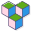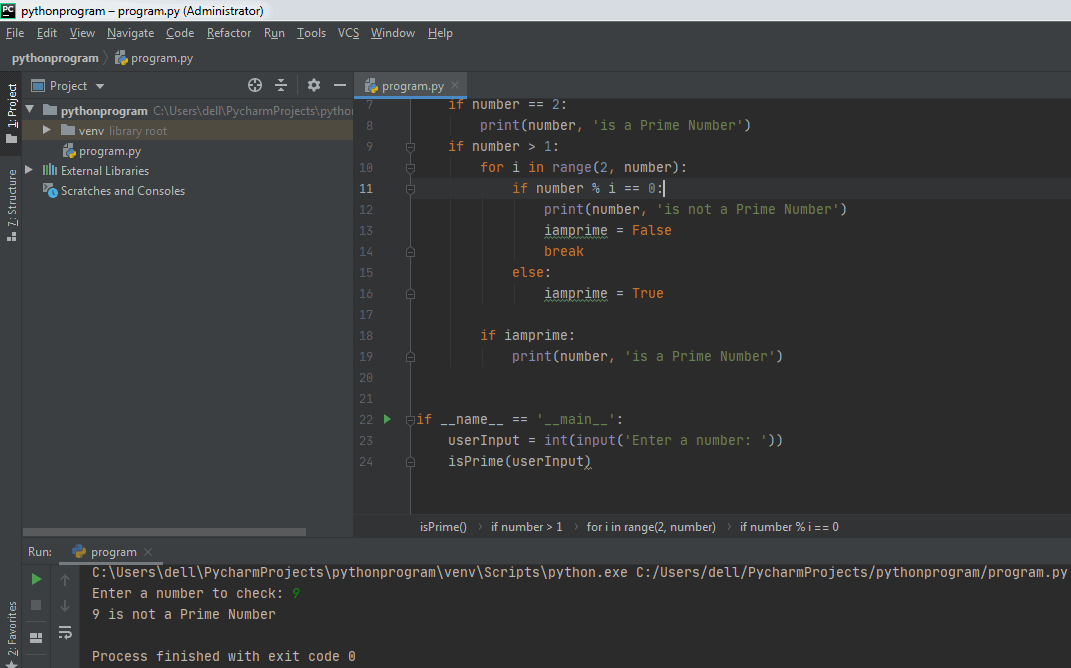CppBuzz# Python program to check if number is prime

This program checks if the entered number is prime or not.

# Author: CppBuzz.com
# This program checks whether the entered number is prime or not

```
def isPrime(number):
"""This function checks for prime number"""
iamprime = False
if number == 2:
print(number, 'is a Prime Number')
if number > 1:
for i in range(2, number):
if number % i == 0:
print(number, 'is not a Prime Number')
iamprime = False
break
else:
iamprime = True

if iamprime:
print(number, 'is a Prime Number')

if __name__ == '__main__':
userInput = int(input('Enter a number: '))
isPrime(userInput)

```